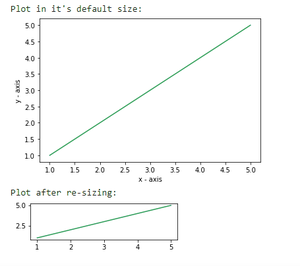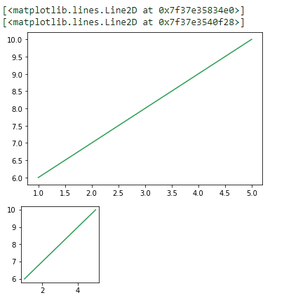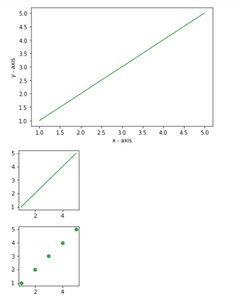GeeksforGeeks App
Open AppBrowser
Continue

# Change plot size in Matplotlib – Python

Prerequisite: Matplotlib

Plots are an effective way of visually representing data and summarizing it in a beautiful manner. However, if not plotted efficiently it seems appears complicated. In python’s matplotlib provides several libraries for the purpose of data representation.

While making a plot it is important for us to optimize its size. Here are various ways to change the default plot size as per our required dimensions or resize a given plot.

Method 1: Using set_figheight() and set_figwidth()

For changing height and width of a plot set_figheight and set_figwidth are used

## Python3

 `# importing the matplotlib library``import` `matplotlib.pyplot as plt`` ` `# values on x-axis``x ``=` `[``1``, ``2``, ``3``, ``4``, ``5``]``# values on y-axis``y ``=` `[``1``, ``2``, ``3``, ``4``, ``5``]`` ` `# naming the x and y axis``plt.xlabel(``'x - axis'``)``plt.ylabel(``'y - axis'``)`` ` `# plotting a line plot with it's default size``print``(``"Plot in it's default size: "``)``plt.plot(x, y)``plt.show()`` ` `# plotting a line plot after changing it's width and height``f ``=` `plt.figure()``f.set_figwidth(``4``)``f.set_figheight(``1``)`` ` `print``(``"Plot after re-sizing: "``)``plt.plot(x, y)``plt.show()`

Output:Method 2: Using figsize

figsize() takes two parameters- width and height (in inches). By default the values for width and height are 6.4 and 4.8 respectively.

Syntax:

plt.figure(figsize=(x,y))

Where, x and y are width and height respectively in inches.

## Python3

 `import` `matplotlib.pyplot as plt`` ` `# values on x and y axis``x ``=` `[``1``, ``2``, ``3``, ``4``, ``5``]``y ``=` `[``6``, ``7``, ``8``, ``9``, ``10``]`` ` `# plot in it's default size``display(plt.plot(x, y))`` ` `# changing the size of figure to 2X2``plt.figure(figsize``=``(``2``, ``2``))``display(plt.plot(x, y))`

Output:output screenshot

Method 3: Changing the default rcParams

We can permanently change the default size of a figure as per our needs by setting the figure.figsize.

## Python3

 `# importing the matplotlib library``import` `matplotlib.pyplot as plt`` ` `# values on x-axis``x ``=` `[``1``, ``2``, ``3``, ``4``, ``5``]``# values on y-axis``y ``=` `[``1``, ``2``, ``3``, ``4``, ``5``]`` ` `# naming the x axis``plt.xlabel(``'x - axis'``)``# naming the y axis``plt.ylabel(``'y - axis'``)`` ` `# plotting a line plot with it's default size``plt.plot(x, y)``plt.show()`` ` `# changing the rc parameters and plotting a line plot``plt.rcParams[``'figure.figsize'``] ``=` `[``2``, ``2``]`` ` `plt.plot(x, y)``plt.show()`` ` `plt.scatter(x, y)``plt.show()`

Output:My Personal Notes arrow_drop_up